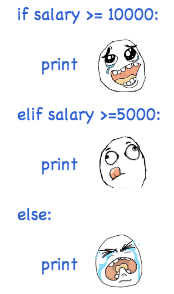> Python3中文手册 > 条件判断

# 条件判断

## 条件判断

``````age = 20
if age >= 18:

``````age = 3
if age >= 18:
else:
print('teenager')``````

``````age = 3
if age >= 18:
elif age >= 6:
print('teenager')
else:
print('kid')``````

`elif``else if`的缩写，完全可以有多个`elif`，所以`if`语句的完整形式就是：

``````if <条件判断1>:
<执行1>
elif <条件判断2>:
<执行2>
elif <条件判断3>:
<执行3>
else:
<执行4>``````

`if`语句执行有个特点，它是从上往下判断，如果在某个判断上是`True`，把该判断对应的语句执行后，就忽略掉剩下的`elif``else`，所以，请测试并解释为什么下面的程序打印的是`teenager`

``````age = 20
if age >= 6:
print('teenager')
elif age >= 18:
else:
print('kid')``````

`if`判断条件还可以简写，比如写：

``````if x:
print('True')``````

## 再议 input

``````birth = input('birth: ')
if birth < 2000:
print('00前')
else:
print('00后')``````

``````Traceback (most recent call last):
File "<stdin>", line 1, in <module>
TypeError: unorderable types: str() > int()``````

``````s = input('birth: ')
birth = int(s)
if birth < 2000:
print('00前')
else:
print('00后')``````

``````Traceback (most recent call last):
File "<stdin>", line 1, in <module>
ValueError: invalid literal for int() with base 10: 'abc'``````

## 练习

• 低于18.5：过轻
• 18.5-25：正常
• 25-28：过重
• 28-32：肥胖
• 高于32：严重肥胖

`if-elif`判断并打印结果：

``````# -*- coding: utf-8 -*-

height = 1.75
weight = 80.5
bmi = ???
if ???:
pass``````

## 小结do_if.py